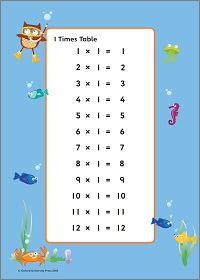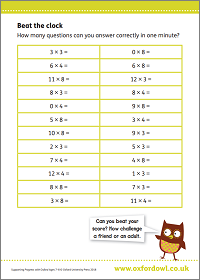We use cookies to enhance your experience on our website. By continuing to use our website, you are agreeing to our use of cookies. You can change your cookie settings at any time. Find out more
0 Items
Select Page

# Multiplication & Division

Understanding how to multiply and divide numbers is essential to be able to solve maths problems quickly. The multiplication and division skills your child learns at primary school will go on to help them in everyday life.

At the end of primary school, children sit mandatory tests in arithmetic and reasoning. Although this might seem a bit daunting, your child build their skills gradually through the primary years. So they will progress from counting in steps of 2, 5, and 10 in Year 1 to using long multiplication and long division with large numbers in Year 6.

There are lots of things you can do at home to support your child’s developing multiplication and division skills.

## What your child will learn

Follow the links below for an overview of each year, with lots of information, support, and practice activities:

#### Multiplication & division in Year 1 (age 5–6)

In Year 1, your child will be expected to be able to solve simple multiplication and division problems using objects, drawings, and arrays to help them. This includes:

• counting in steps of 2, 5, and 10 and understanding that, for example, 3 × 2 is the same as 2 + 2 + 2
• sharing and grouping to solve division problems
• beginning to understand the relationship between multiplication and division.

#### Multiplication & division in Year 2 (age 6–7)

In Year 2, your child will be expected to use a range of methods to solve multiplication and division problems, including using practical resources and mental methods. This includes:

• knowing and using multiplication and division facts for the 2, 5, and 10 times tables
• recognising and identifying odd and even numbers
• using the symbols ×, ÷, and = to record multiplication and division calculations.

#### Multiplication & division in Year 3 (age 7–8)

In Year 3, your child will be expected to use a range of strategies to solve problems mentally and will begin to learn formal written methods for short multiplication and short division. This includes:

• knowing and using multiplication and division facts for the 3, 4, and 8 times tables
• multiplying two-digit numbers by one-digit numbers
• understanding that multiplication and division have an inverse relationship (i.e. they undo each other), and using this to check their calculations.

#### Multiplication & division in Year 4 (age 8–9)

In Year 4, your child will be expected to be able to use formal written methods of short multiplication and short division confidently. This includes:

• knowing and using multiplication and division facts for all times tables up to 12 × 12
• multiplying three-digit numbers by one-digit numbers
• multiplying three numbers together
• preparing for the Year 4 multiplication tables check in June.

#### Multiplication & division in Year 5 (age 9–10)

In Year 5, your child will be expected to be able to solve multiplication and division problems involving numbers up to four digits and begin to learn long multiplication. This includes:

• multiplying four-digit numbers by two-digit numbers
• dividing four-digit numbers by one-digit numbers and interpreting remainders
• understanding the terms multiple, factor, common factor, prime, square, and cube numbers.

#### Calculation in Year 6 (age 10–11)

In Year 6, your child will be expected to be able to multiply and divide with large numbers using formal written methods including long division. This includes:

• multiplying four-digit numbers by two-digit numbers using long multiplication
• dividing four-digit numbers by two-digit numbers using long division
• solving multi-step problems using addition, subtraction, multiplication, and division choosing which methods to use and explaining why.

## How to help at home

You don’t need to be an expert to support your child with maths! Here are three simple but effective ways to help your child develop their understanding of multiplication and division.

### 1. Explore practically

Use real objects to develop early understanding of multiplication and division. For example, use socks, gloves or ice-cube trays to count in twos, fives, or tens. Use egg boxes or muffin trays to explore arrays. Practise division by sharing beads between toys or arranging blocks into groups.

### 2. Practise times tables

Sing, chant or play games to help your child to memorise times tables. Give points for each fact they know. Use real-life opportunities to practise. For example, when you’re in the supermarket ask your child: ‘How many packets will we have if we buy 3 multipacks with 6 packets in each?’

### Activity: Times tables wall chartsPrint-out charts for all times tables from 1–12.

### Activity: Beat the clockAnswer as many as you can in one minute.

## 3. Explain different methods

Ask your child to explain each stage of a multiplication or division and why they chose that method. They might use doubling or halving, apply times tables facts, pictures to represent their calculation, or written methods. Encourage them to estimate first and then check with a different strategy.

## Video support

### What is the grid method of multiplication?

Find out what grid multiplication is and discover fun ways to practise multiplication with your child from mathemagician Andrew Jeffrey.

### How to do long division

Homework help! Find out how to divide numbers using long division.

## Maths glossary

Use these quick links or explore our jargon buster for simple definitions and examples of mathematical terms.

• Area/grid method is a way of visualising multiplication, as a first step towards formal written calculations, where numbers are set out in a grid.
• Arrays are shapes or objects arranged in a rectangle. For example, egg boxes and muffin trays are arrays.
• Common factors are numbers that can be divided into two different numbers. For example, the common factors of 12 and 18 are 1, 2, 3, and 6 because they all divide into both 12 and 18.
• Factors are whole numbers that will divide exactly into another number. For example 1, 2, 3, 4, 6 and 12 are all factors of 12.
• Inverse operations means that we can use one operation to ‘undo’ the other. For example, multiplication is the inverse of division.

Search our education glossary >